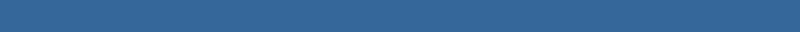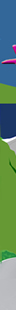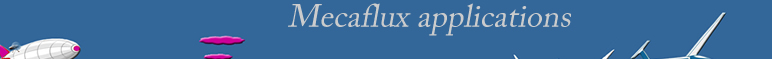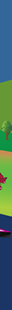For a more precise evaluation of the hydrodynamic drag hull shape of a ship or submarine, the HELICIEL software allows you to choose a hydrodynamic profile and calculate its performance drag. then export the 3D geometry for a design with CAD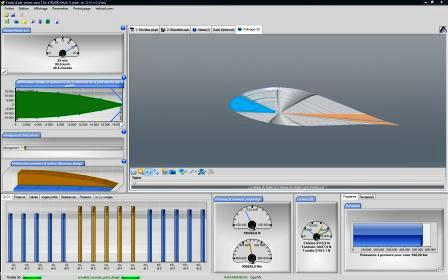DRAG calculation drag coefficient Cd

The drag force can be calculated from:

with the drag formula:

p is the density of the fluid KG/m3

S front surface or Wing area  m²

v the relative velocity* of the fluid m/s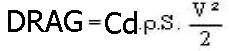*whether the fluid, the object, or both moving, we take into account the speed relative to each other..

Cd and Cl (drag and lift coefficient) measured experimentally are in the database, where the value of disks is always given as a function of the Reynolds number, which includes the fluid kinematic viscosity.

Methods or mathematical determination of Cd Cl exist, reaching levels of interesting information. The coefficients of drag and lift are often represented by graphs called Polars.

The drag force is expressed in Newtons, It is that which resists movement or slows down the ship.

The CD allows us to extrapolate the resistance to progress for a series of geometrically similar profiles.

When the drag is studied in water we talk about viscous resistance: Rv and the formula for calculating the coefficient of viscous drag is related to the Reynolds number Re and takes the name of viscous drag coefficient: Cv

Rv= Cv . (Re . K/L).

rugosity(K)

In naval hydrodynamics, sometimes a method method of dual models are used to determine the viscous resistance.

With MECAFLUX standard

Detail of the interface drag and lift calculation in MECAFLUX standard